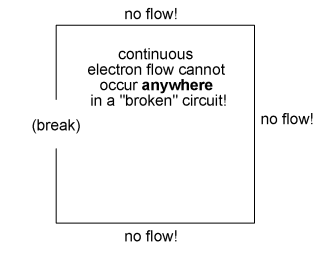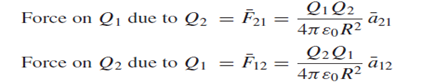Voltage and current:: Voltage attempts to make a current flow, and current will flow if the circuit is complete. Voltage is sometimes described as the 'push' or 'force' of the electricity, it isn't really a force but this may help you to imagine what is happening. It is possible to have voltage without current, but current cannot flow without voltage.

Charge: electric charge, basic property of matter carried by some elementary particles. Electric charge, which can be positive or negative, occurs in discrete natural units and is neither created nor destroyed.

Electric circuits: which are collections of circuit elements connected together, are the most fundamental structures of electrical engineering. A circuit is an interconnection of simple electrical devices that have at least one closed path in which current may flow. However, we may have to clarify to some of our readers what is meant by “current” and “electrical device,” a task that we shall undertake shortly. Circuits are important in electrical engineering because they process electrical signals, which carry energy and information; a signal can be any time varying electrical quantity. Engineering circuit analysis is a mathematical study of some useful interconnection of simple electrical devices.

In describing the operation of electric circuits, one should be familiar with such electrical quantities as charge, current, and voltage.Charge and Electric Force: The proton has a charge of 1.602 10−19 coulombs (C), while the electron has a charge of −1.602 × 10−19 C. The neutron has zero charge. Electric charge and, more so, its movement are the most basic items of interest in electrical engineering. When many charged particles are collected together, larger charges and charge distributions occur. There may be point charges (C), line charges (C/m), surface charge distributions (C/m2), and volume charge distributions (C/m3).

Coulomb’s law gives an expression to evaluate the electric force in newtons (N) exerted on one point charge by the other:where Q1 and Q2 are the point charges (C); R is the separation in meters (m) between them; ε0

is the permittivity of the free-space medium with units of C2/N.